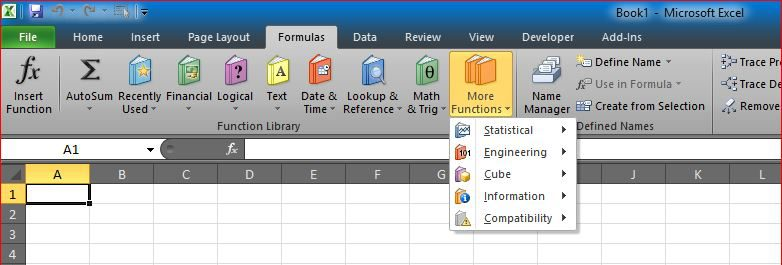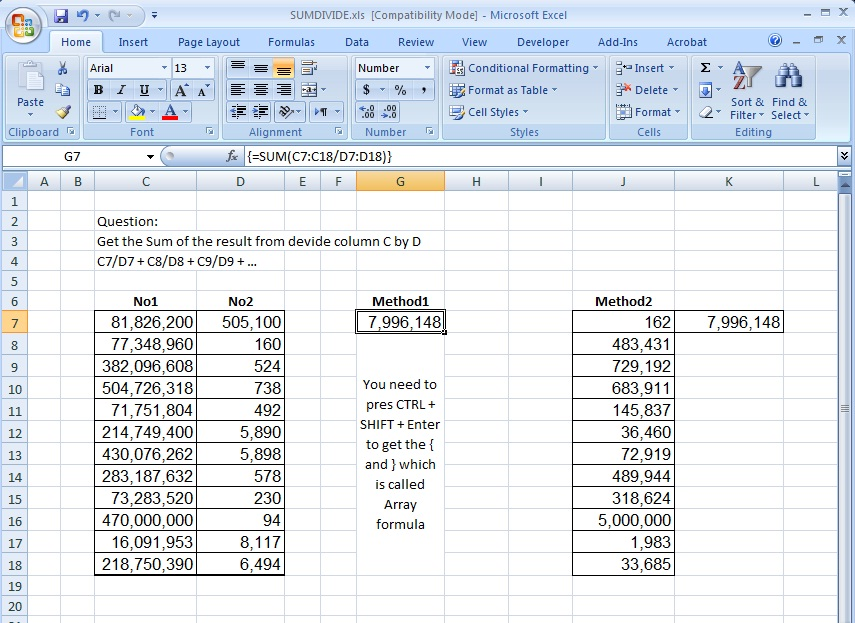# Sum of divide – Array formulaThis is the answer to an old question:

Here is the story behind it:

I had a client who calls me every now and then asking the same question:

” Can you please remind me of how I would use an array formula? you know, enter multiple conditions into one SUM function? ”

Then I go a head and show him the procedure and the result, most of the time he uses phone, so I have to take him with the steps needed.

Now after these years, I found the xls file in my library, thought it is going to be good to share it here.

It is the one that divide then Sum the totals into one cell (which uses array formula to do that)

Formulas used here are:

`=SUM(C7:C18/D7:D18)`

In cell G7 (Remember to press CTRL+SHIFT+Enter after pasting to apply the Array formula)

Above formula is equivalent to two formulas, one in column J:

`=C7/D7`

And another one in cell K7

`=SUM(J:J)`